# Understanding regularization for image classification and machine learning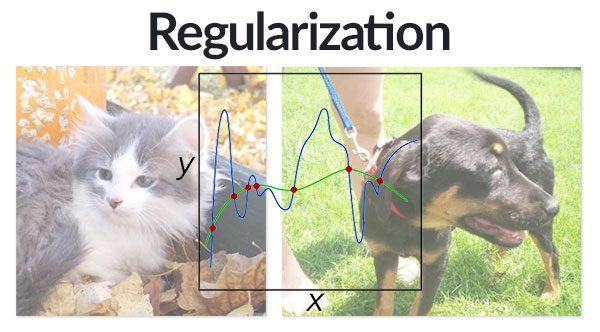In previous tutorials, I’ve discussed two important loss functions: Multi-class SVM loss and cross-entropy loss (which we usually refer to in conjunction with Softmax classifiers).

In order to to keep our discussions of these loss functions straightforward, I purposely left out an important component: regularization.

While our loss function allows us to determine how well (or poorly) our set of parameters (i.e., weight matrix, and bias vector) are performing on a given classification task, the loss function itself does not take into account how the weight matrix “looks”.

What do  I mean by “looks”?

Well, keep in mind that there may be an infinite set of parameters that obtain reasonable classification accuracy on our dataset — how do we go about choosing a set of parameters that will help ensure our model generalizes well? Or at the very least, lessen the affects of overfitting?

There are various types of regularization techniques, such as L1 regularization, L2 regularization, and Elastic Net — and in the context of Deep Learning, we also have dropout (although dropout is more-so a technique rather than an actual function).

Inside today’s tutorial, we’ll mainly be focusing on the former rather than the later. Once we get to more advanced deep learning tutorials, I’ll dedicate time to discussing dropout as well.

In the remainder of this blog post, I’ll be discussing regularization further. I’ll also demonstrate how to update our Multi-class SVM loss and cross-entropy loss functions to include regularization. Finally, we’ll write some Python code to construct a classifier that applies regularization to an image classification problem.

Looking for the source code to this post?

## Understanding regularization for image classification and machine learning

The remainder of this blog post is broken into four parts. First, we discuss what regularization is. I then detail how to update our loss function to include the regularization term.

From there, I list out three common types of regularization you’ll likely see when performing image classification and machine learning, especially in the context of neural networks and deep learning.

Finally, I’ll provide a Python + scikit-learn example that demonstrates how to apply regularization to an image classification dataset.

### What is regularization and why do we need it?

Regularization helps us tune and control our model complexity, ensuring that our models are better at making (correct) classifications — or more simply, the ability to generalize.

If we don’t apply regularization, our classifiers can easily become too complex and overfit to our training data, in which case we lose the ability to generalize to our testing data (and data points outside the testing set as well).

Similarly, without applying regularization we also run the risk of underfitting. In this case, our model performs poorly on the training our — our classifier is not able to model the relationship between the input data and the output class labels.

Underfitting is relatively easy to catch — you examine the classification accuracy on your training data and take a look at your model.

If your training accuracy is very low and your model is excessively simple, then you are likely a victim of underfitting. The normal remedy to underfitting is to essentially increase the number of parameters in your model, thereby increasing complexity.

Overfitting is a different beast entirely though.

While you can certainly monitor your training accuracy and recognizing when your classifier is performing too well on the training data and not good enough on the testing data, it becomes harder to correct.

There is also the problem that you can walk a very fine line between model complexity — if you simplify your model too much, then you’ll be back to underfitting.

A better approach is to apply regularization which will help our model generalize and lead to less overfitting.

The best way to understand regularization is to see the implications it has on our loss function, which I discuss in the next section.

### Updating our loss function to include regularization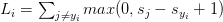$L_{i} = \sum_{j \neq y_{i}} max(0, s_{j} - s_{y_{i}} + 1)$

The loss for the entire training set can be written as: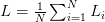$L = \frac{1}{N} \sum^{N}_{i=1} L_{i}$

Now, let’s say that we have obtained a weight matrix W such that every data point in our training set is classified 100% correctly — this implies that our loss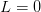$L = 0$ for all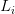$L_{i}$.

Awesome, we’re getting 100% accuracy — but let me ask you a question about this weight matrix W — is this matrix unique?

Or in other words, are there BETTER choices of W that will improve our model’s ability to generalize and reduce overfitting?

If there is such a W, how do we know? And how can we incorporate this type of penalty into our loss function?

The answer is to define a regularization penalty, a function that operates on our weight matrix W.

The regularization penalty is commonly written as the function R(W).

Below is the most common regularization penalty, L2 regularization: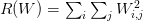$R(W) = \sum_{i}\sum_{j} W_{i,j}^{2}$

What is this function doing exactly?

To answer this question, if I were to write this function in Python code, it would look something like this using two for  loops:

What we are doing here is looping over all entries in the matrix and taking the sum of squares. There are more efficient ways to compute this of course, I’m just simplifying the code as a matter of explanation.

The sum of squares in the L2 regularization penalty discourages large weights in our weight matrix W, preferring smaller ones.

Why might we want to discourage large weight values?

In short, by penalizing large weights we can improve our ability to generalize, and thereby reduce overfitting.

Think of it this way — the larger a weight value is, the more influence it has on the output prediction. This implies that dimensions with larger weight values can almost singlehandedly control the output prediction of the classifier (provided the weight value is large enough, of course), which will almost certainly lead to overfitting.

To mitigate the affect various dimensions have on our output classifications, we apply regularization, thereby seeking W values that take into account all of the dimensions rather than the few with large values.

In practice, you may find that regularization hurts your training accuracy slightly, but actually increases your testing accuracy (your ability to generalize).

Again, our loss function has the following basic form, but now we just add in regularization: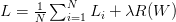$L = \frac{1}{N} \sum^{N}_{i=1} L_{i} + \lambda R(W)$

The first term we have already seen before — this is the average loss over all samples in our training set.

The second term is new — this is our regularization term.

The$\lambda$ variable is a hyperparameter that controls the amount or strength of the regularization we are applying. In practice, both the learning rate$\alpha$ and regularization term$\lambda$ are hyperparameters that you’ll spend most of your time tuning.

Expanding the Multi-class SVM loss to include regularization yields the final equation: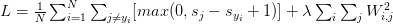$L =\frac{1}{N} \sum^{N}_{i=1} \sum_{j \neq y_{i}} [max(0, s_{j} - s_{y_{i}} + 1)] + \lambda \sum_{i} \sum_{j} W_{i, j}^{2}$

We can also expand cross-entropy loss in a similar fashion: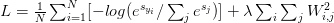$L =\frac{1}{N} \sum^{N}_{i=1} [-log(e^{s_{y_{i}}} / \sum_{j} e^{s_{j}})] +\lambda \sum_{i} \sum_{j} W_{i, j}^{2}$

For a more mathematically motivated discussion of regularization, take a look at Karpathy’s excellent slides from the CS231n course.

### Types of regularization techniques

In general, you’ll see three common types of regularization.

The first, we reviewed earlier in this blog post, L2 regularization;$R(W) = \sum_{i}\sum_{j} W_{i,j}^{2}$

We also have L1 regularization which takes the absolute value rather than the square: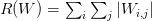$R(W) = \sum_{i}\sum_{j} |W_{i,j}|$

Elastic Net regularization seeks to combine both L1 and L2 regularization: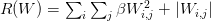$R(W) = \sum_{i}\sum_{j} \beta W_{i,j}^{2} + |W_{i,j}|$

In terms of which regularization method you should be using (including none at all), you should treat this choice as a hyperparameter you need to optimize over and perform experiments to determine if regularization should be applied, and if so, which method of regularization.

Finally, I’ll note that there is another very common type of regularization that we’ll see in a future tutorial — dropout.

Dropout is frequently used in Deep Learning, especially with Convolutional Neural Networks.

Unlike L1, L2, and Elastic Net regularization, which boil down to functions defined in the form R(W), dropout is an actual technique we apply to the connections between nodes in a Neural Network.

As the name implies, connections “dropout” and randomly disconnect during training time, ensuring that no one node in the network becomes fully responsible for “learning” to classify a particular label. I’ll save a more thorough discussion of dropout for a future blog post.

### Image classification using regularization with Python and scikit-learn

Now that we’ve discussed regularization in the context of machine learning, let’s look at some code that actually performs various types of regularization.

All of the code associated with this blog post, expect for the final code block, has already been reviewed extensively in previous blog posts in this series.

Therefore, for a thorough review of the actual process used to extract features and construct the training and testing split for the Kaggle Dogs vs. Cats dataset, I’ll refer you to the introduction to linear classification tutorial.

The code block below demonstrates how to apply the Stochastic Gradient Descent (SGD) classifier with log-loss (i.e., Softmax) and various types of regularization methods to our dataset:

On Line 74 we start looping over our regularization methods, including None  for no regularization.

We then train our SGDClassifier  on Lines 78-80 using the specified regularization method.

Lines 83 and 84 evaluate our trained classifier on the testing data and display the accuracy.

Below I have included a screenshot from executing the script on my machine: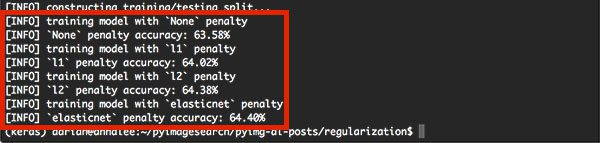Figure 1: Applying no regularization, L1 regularization, L2 regularization, and Elastic Net regularization to our classification project.

As we can see, classification accuracy on the testing set improves as regularization is introduced.

We obtain 63.58% accuracy with no regularization. Applying L1 regularization increases our accuracy to 64.02%. L2 regularization improves again to 64.38%. Finally, Elastic Net, which combines both L1 and L2 regularization obtains the highest accuracy of 64.40%.

Does this mean that we should always apply Elastic Net regularization?

Of course not — this is entirely dependent on your dataset and features. You should treat regularization, and any parameters associated with your regularization method, as hyperparameters that need to be searched over.

## Summary

In today’s blog post, I discussed the concept of regularization and the impact it has on machine learning classifiers. Specifically, we use regularization to control overfitting and underfitting.

Regularization works by examining our weight matrix W and penalizing it if it does not confirm to the specified penalty function.

Applying this penalty helps ensure we learn a weight matrix W that generalizes better and thereby helps lesson the negative affects of overfitting.

In practice, you should apply hyperparameter tuning to determine:

1. If regularization should be applied, and if so, which regularization method should be used.
2. The strength of the regularization (i.e., the$\lambda$ variable).

You may notice that applying regularization may actually decrease your training set classification accuracy — this is acceptable provided that your testing set accuracy increases, which would be a demonstration of regularization in action (i.e., avoiding/lessening the impact of overfitting).

In next week’s blog post, I’ll be discussing how to build a simple feedforward neural network using Python and Keras. Be sure to enter your email address in the form below to be notified when this blog post goes live!If you would like to download the code and images used in this post, please enter your email address in the form below. Not only will you get a .zip of the code, I’ll also send you a FREE 17-page Resource Guide on Computer Vision, OpenCV, and Deep Learning. Inside you'll find my hand-picked tutorials, books, courses, and libraries to help you master CV and DL! Sound good? If so, enter your email address and I’ll send you the code immediately!

### 6 Responses to Understanding regularization for image classification and machine learning

1.Ashis Samal September 20, 2016 at 1:42 pm #

Thank you very much.Nice explanation .

•Adrian Rosebrock September 21, 2016 at 2:11 pm #

2.Manuel May 25, 2017 at 3:40 pm #

Hi Adrian, a question regarding the search for the best regularization term using the techniques you explain in your “How to tune hyperparameters with Python and scikit-learn” post.

How should I specify in the RandomizedSearchCV function, that the score I want to maximize is the accuracy on the validation set and not the one of the train set?

Thanks!

•Manuel May 25, 2017 at 4:06 pm #

Wait, I think that CV at the end of the function means cross-validation, so accuracy report is already being done on a cross-validated set, which would be equivalent to report on a validation set, right?

•Adrian Rosebrock May 28, 2017 at 1:22 am #

The cross-validation will be performed by dividing your training set into N parts, training on all but one of the sets, and then validating on the other. This is done for each data split and serves as a proxy for your validation.

3.Igor June 15, 2018 at 12:55 pm #

I understood that regularization helps to fix the overfitting. But, you said “… without applying regularization we also run the risk of underfitting.” Why is that? Regulariztion makes the network simpler to avoid overfitting, so how does regulariztion help with underfitting?